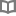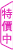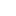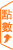你一定想看的韓國史 - 楊益、鄭嘉偉 - BOOKWALKER中文電子書

你一定想看的韓國史

楊益、鄭嘉偉

作者簡介 楊益 曾經從事過高中教師、漫畫文字編輯、網站編輯、IT產品設計等工作，兼任廣播電台頻道嘉賓和晚報專欄撰稿人，已出版《張居正全傳》、《那些三國的女人們》、《成功銷售要讀三國》、《不可不知的朝韓史》、《諸葛亮全傳》、《張騫出使西域》、《人類社會的神經系統》、《通信原理》、《宋國的故事》。 鄭嘉偉 喜歡翻看各種歷史書，閱讀先賢們流傳下來的故事，沉溺於書中的世界而不能自拔，目前從事與圖書相關的工作，希望透過自己的努力，與更多人分享閱讀的樂趣。

• 放入購物車
•試閱

• 放入購物車
•試閱• 放入購物車
•試閱

• 放入購物車
•試閱

• 放入購物車
•無試閱

• 放入購物車
•試閱

• 放入購物車
•試閱

• 放入購物車
•試閱• 放入購物車
•試閱• 放入購物車
•試閱

• 放入購物車
•試閱

• 放入購物車
•試閱

• 放入購物車
•試閱• 放入購物車
•試閱

• 放入購物車
•試閱

• 放入購物車
•試閱• 放入購物車
•試閱

• 放入購物車
•試閱

• 放入購物車
•試閱

• 放入購物車
•試閱

• 放入購物車
•試閱

• 放入購物車
•試閱

• 放入購物車
•試閱

• 放入購物車
•試閱

• 放入購物車
•試閱

• 放入購物車
•試閱

• 放入購物車
•試閱

• 放入購物車
•試閱

• 放入購物車
•試閱• 放入購物車
•試閱• 放入購物車
•試閱• 放入購物車
•無試閱

• 放入購物車
•試閱

• 放入購物車
•試閱

• 放入購物車
•試閱

• 放入購物車
•試閱

• 放入購物車
•試閱

• 放入購物車
•試閱

• 放入購物車
•試閱

• 放入購物車
•試閱

• 放入購物車
•試閱

• 放入購物車
•試閱

• 放入購物車
•試閱

• 放入購物車
•無試閱• 放入購物車
•試閱

• 放入購物車
•試閱

• 放入購物車
•試閱